# What is the Order of Operations in Math (PEMDAS)?

Does the order in which we solve math problems matter? Yes! Let’s learn about the order of operations in math, aka PEMDAS, to solve multi-step expressions.Author
Amber WatkinsPublished
November 7, 2023# What is the Order of Operations in Math (PEMDAS)?

Does the order in which we solve math problems matter? Yes! Let’s learn about the order of operations in math, aka PEMDAS, to solve multi-step expressions.Author
Amber WatkinsPublished
Nov 7, 2023# What is the Order of Operations in Math (PEMDAS)?

Does the order in which we solve math problems matter? Yes! Let’s learn about the order of operations in math, aka PEMDAS, to solve multi-step expressions.Author
Amber WatkinsPublished
Nov 7, 2023Key takeaways

• The order of operations teaches the order in which to solve multi-operational expressions.
• The order of operations can easily be remembered using the acronym PEMDAS.
• As your child successfully masters the order of operations, they will be able to solve more advanced problems with ease.

## What is the Order of Operations in Math (PEMDAS)?When reading a math problem, we usually start from left to right. So it is natural to want to solve problems in the same way. Does this method get us the right answer for every problem?

Let’s solve the following problem in two ways to see how order makes a difference: 3 – 2 x 5 =

1. You can solve this problem by subtracting first.

3 – 2 x 5

1 x 5

5

2. Or you can solve it by multiplying first.

3 – 2 x 5

3 – 10

-7

In both examples, we took the same actions: we multiplied and subtracted, but switched the order. As a result, we found two very different answers. How can we know which is the right answer? By following the math order of operations.

## What is the Order of Operations in Math?Operations are the actions we take in math. The most common math operations are adding, subtracting, multiplying, dividing, simplifying exponents, and finding square roots.

Some math problems have more than one kind of operation in the problem. How can we solve those types of problems? We follow the order of operations!

What is the order of operations? The order of operations tells us the order in which to perform operations when the problem contains more than one kind of operation.

## Order of Operations RulesMany times you will see the order of operations called PEMDAS. What is Pemdas? Pemdas is an acronym that helps us remember the order in which to solve multi-operational problems.

What does Pemdas stand for? PEMDAS is an acronym that stands for Parenthesis, Exponents, Multiplication or Division, Adding or Subtracting.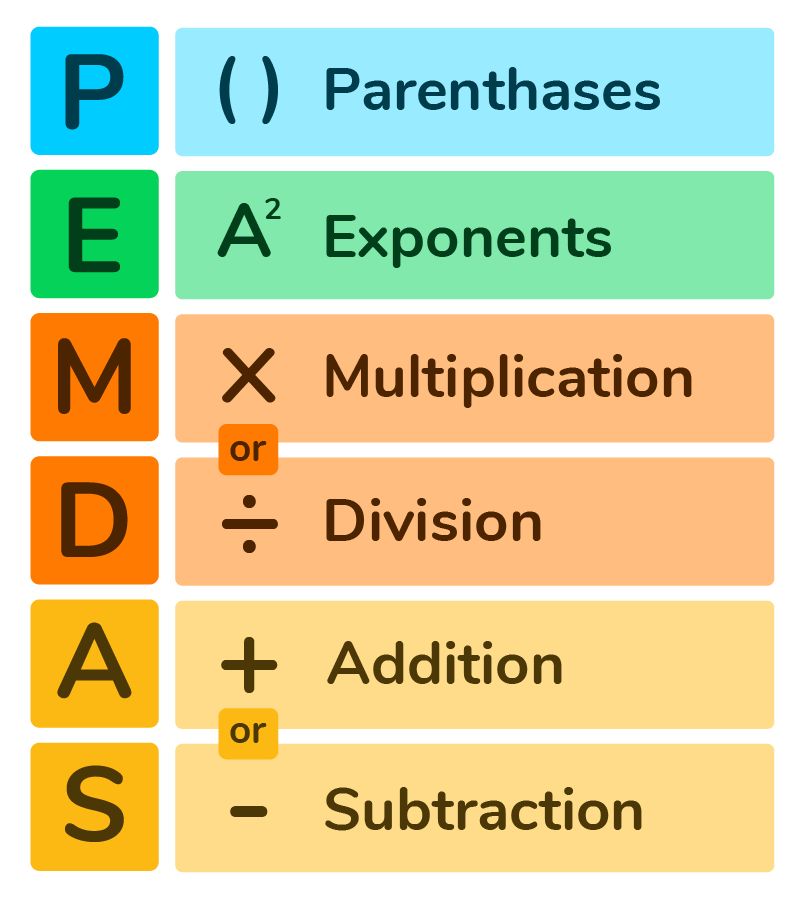Now that we know the Pemdas meaning, let’s discuss the Pemdas Rule.

#1 PEMDAS Rule – When solving order of operation problems in math, you must follow the order of the acronym.

First, you solve whatever operations are in the parenthesis. ()

Second, you calculate any exponents.

Third, you multiply or divide. x  or  ÷

Fourth, you add or subtract. + or –

## BODMAS vs PEMDASYou may also hear the order of operations called BODMAS. What is BODMAS?

BODMAS stands for Brackets, Orders (Powers or Roots), Division or Multiplication, Adding or Subtracting.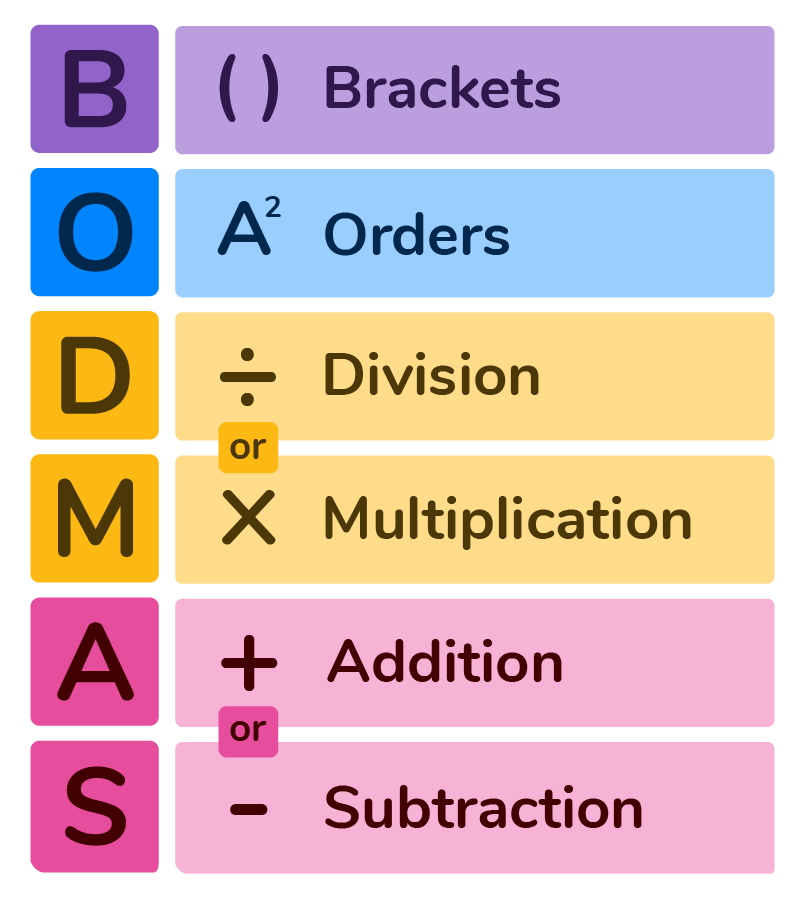Brackets are another name for parenthesis and orders are another name for exponents. Although the terms used for operations are different than Pemdas, both Bodmas and Pemdas follow the same rules of order of operations.

What are those rules and how can we use them to solve PEMDAS problems?

## How to Solve Order of Operations ProblemsLet’s solve this Order of Operations problem as we learn some PEMDAS rules.

4² – ( 3 x 5 ) + 9 ፥ 3 x 2

1. Pemdas Rule for Parenthesis
When solving order of operation problems, first complete the operations that are found inside the parenthesis or brackets.

In our example problem, we would multiply 3 x 5 first because it is in the parenthesis.

P
E M D A S

4² – ( 3 x 5 ) + 9 ፥ 3 x 2

4² – 15 + 9 ፥ 3 x 2

2. Pemdas Rule for Exponents

Exponents should always be simplified before moving to the next step in the order of operations. If you begin multiplying, dividing, adding, or subtracting while there is still an exponent in the problem, you may have missed this step.

This is how we would simplify the exponents.

P E M D A S

– 15 + 9 ፥ 3 x 2

16 – 15 + 9 ፥ 3 x 2

3. Pemdas Rule for Multiplying or Dividing

When using the order of operations, you may have noticed the word “or” is used when multiplying or dividing. When following the order of operations in math, you can multiply or divide in any order. Simply complete the operation that comes first.

We have both multiplication and division in this problem, so we will go from left to right. First is division, then is multiplication.

P E M D A S

16 – 15 + 9 ፥ 3 x 2

16 – 15 + 3 x 2

16 – 15 + 6

4. Pemdas Rule for Adding or Subtraction

When using the order of operations, you may have also noticed the word “or” is used when adding or subtracting. When following the order of operations in math, you can add or subtract in any order. Simply complete the operation that comes first.

Finally, we would add or subtract depending on which operation comes first.

PEMDAS

16 – 15 + 6

1 + 6

7

Now that we know some of the PEMDAS rules, let’s go over some PEMDAS examples together.

## PEDMAS ExamplesPEMDAS EXAMPLE 1: Solve 3 + ( 8 x 9 ) =

Explanation: First, we see parenthesis comes first in the PEMDAS acronym. So we would multiply 8 x 9 because it is in the parenthesis. Therefore, 8 x 9 is equal to 72. Then we would add 3 and 72 to get 75 as our answer.

Our Work for this PEMDAS example would look like this:

3 + ( 8 x 9 )
3 + 72
75

PEMDAS EXAMPLE 2:
Solve 12  ፥ 3 + 14 =

First, we see that division comes before adding in PEMDAS. So we divide 12 by 3 which is equal to 4. Next, add 4 and 14 which equals 18.

Our Work for this PEMDAS example would look like this:

12 ፥ 3 + 14
4 + 14
18

## PEDMAS Practice Questions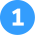1. Use Pemdas to solve 2² + 12 – ( 3 x 2) =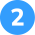2. Use Pemdas to Solve (3 x 2) + (12 ፥ 3 ) =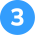3. Use Pemdas to Solve 100  ፥  10 – (6 ፥ 2 ) =First, multiply 3 x 2 because it is in the parenthesis. Then, simplify the exponent 2² which equals 4. Next, add 4 + 12 which equals 16. Finally, subtract 6 which equals 10.

2² + 12 – (3 x 2) =
+ 12 – 6 =
4  + 12 – 6 =
16 – 6 =
10First, multiply 3 x 2 because it is in the first parenthesis. Next, divide 12 by 3 because it is in the second parenthesis. Finally, add 6 + 4 which equals 10.

(3 x 2) + (12 ፥ 3 )
6 + (12 ፥ 3 )
6 + 4
10First, divide 6 by 2 because it is in the parenthesis. Next, divide 100 by 10. Finally, subtract 3 from 10 which equals 7.

100  ፥  10 – (6 ፥ 2 )
100  ፥  10 – 3
10 – 3
7

## FAQs About Order of OperationsPemdas stands for Parenthesis, Exponents, Multiplication or Division, Adding or Subtracting.

In PEMDAS problems, multiplication or division can happen in any order. The Pemdas rule is to complete whichever operation comes first.

In Pemdas problems, always complete whichever operation is inside of the parenthesis first.

BODMAS stands for Brackets, Orders, Division or Multiplication, Adding or Subtracting.

Yes. PEMDAS and BODMAS are both acronyms to represent the order of operations, but they use different words to describe the same operation. For example, PEMDAS uses the terms parenthesis and exponents. BODMAS uses the terms brackets and powers.Lesson credits

Amber Watkins

Amber is an education specialist with a degree in Early Childhood Education. She has over 12 years of experience teaching and tutoring elementary through college level math. "Knowing that my work in math education makes such an impact leaves me with an indescribable feeling of pride and joy!"

Amber Watkins

Amber is an education specialist with a degree in Early Childhood Education. She has over 12 years of experience teaching and tutoring elementary through college level math. "Knowing that my work in math education makes such an impact leaves me with an indescribable feeling of pride and joy!"# Are you a parent, teacher or student?

Are you a parent or teacher?

## Hi there!

Book a chat with our team